### Home > PC > Chapter 4 > Lesson 4.1.1 > Problem4-10

4-10.
1. Copy and replace the question mark with the correct number, or state “impossible” and explain why. Homework Help ✎

1. log3(9) = ?

2. log3 (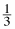) = ?

3. log5(625) = ?

4. log3(0) = ?

5. log5(?) = 2

6. log5(?) =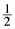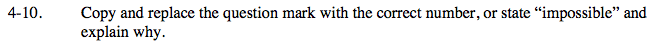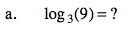It is 2 because 32 = 9.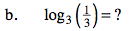$\scriptsize{\frac{1}{3}=3^{-1}}$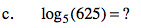5? = 625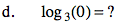3? = 0

Impossible!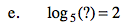52 = ?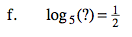51/2 = ?